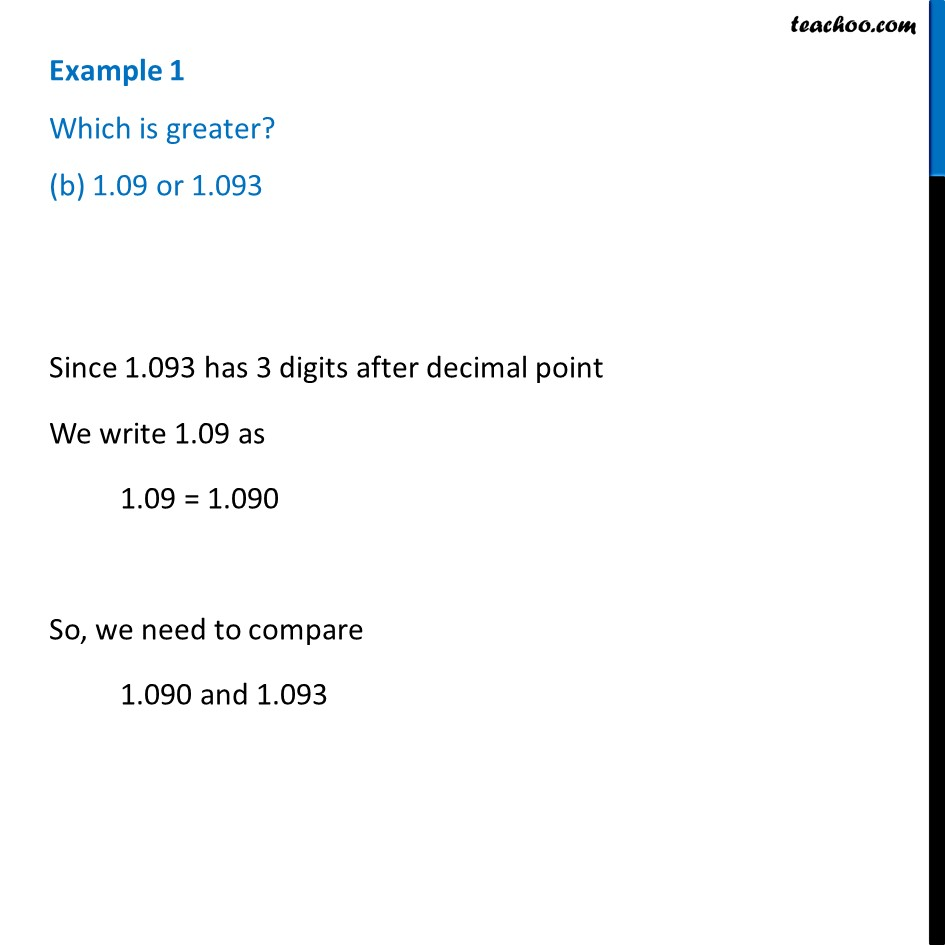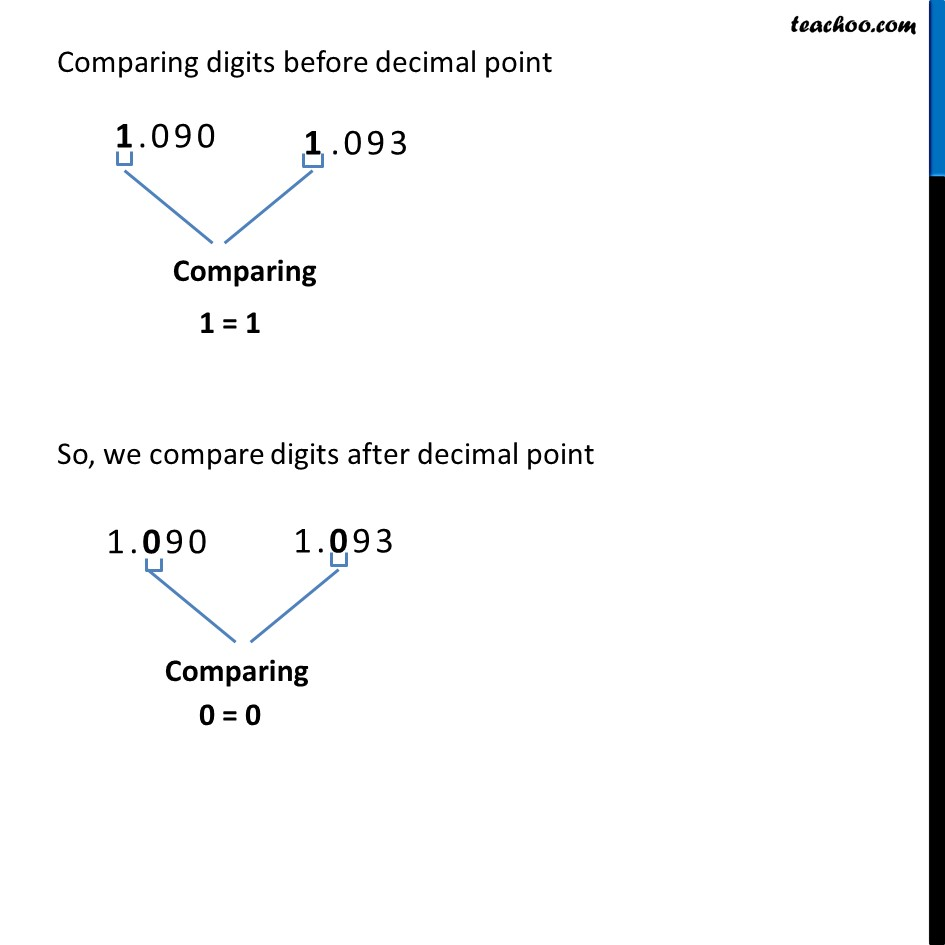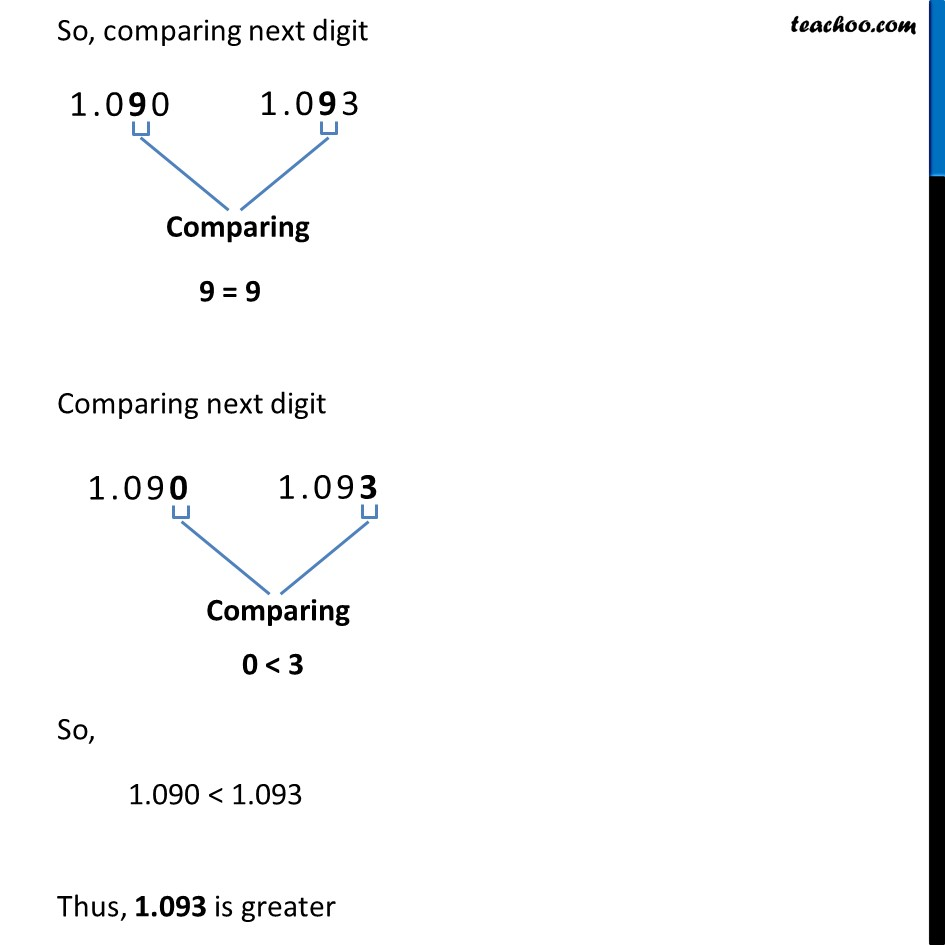Examples

Chapter 8 Class 6 Decimals
Serial order wiseLearn in your speed, with individual attention - Teachoo Maths 1-on-1 Class

### Transcript

Example 1 Which is greater? (b) 1.09 or 1.093 Since 1.093 has 3 digits after decimal point We write 1.09 as 1.09 = 1.090 So, we need to compare 1.090 and 1.093 Comparing digits before decimal point 1 = 1 So, we compare digits after decimal point 0 = 0 So, comparing next digit 9 = 9 Comparing next digit 0 < 3 So, 1.090 < 1.093 Thus, 1.093 is greater